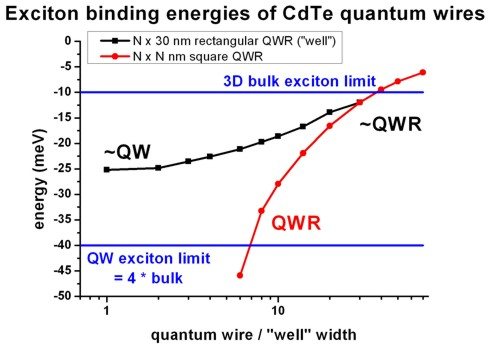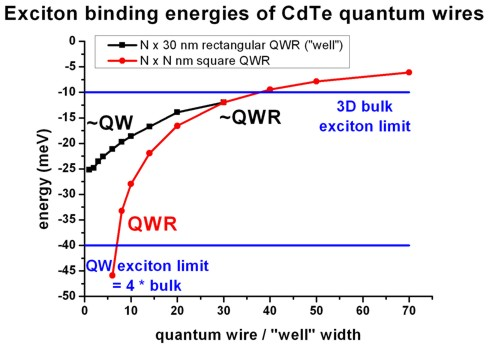nextnano.com  nextnano³  Download | Search | Copyright | Publications  * password protected nextnano³ software2D Exciton in QWR

# nextnano3 - Tutorial

## Exciton energy in quantum wires

Author: Stefan Birner

`-> 2Dwire_excitonCdTe_10x10nm.in  -   10 nm x 10` nm CdTe quantum wire
```   2Dwire_excitonCdTe_14x14nm.in  -   14 nm x 14``` nm CdTe quantum wire
```   2Dwire_excitonCdTe_20x20nm.in  -   20 nm x 20``` nm CdTe quantum wire
```   2Dwire_excitonCdTe_30x30nm.in  -   30 nm x 30``` nm CdTe quantum wire
```   2Dwire_excitonCdTe_40x40nm.in  -   40 nm x 40``` nm CdTe quantum wire
```   2Dwire_excitonCdTe_50x50nm.in  -   50 nm x 50``` nm CdTe quantum wire
```   2Dwire_excitonCdTe_70x70nm.in  -   70 nm x 70``` nm CdTe quantum wire
```-> 2Dwire_excitonCdTe_1x30nm.in   -    1 nm x 30``` nm CdTe quantum wire
```   2Dwire_excitonCdTe_2x30nm.in   -    2 nm x 30``` nm CdTe quantum wire
```   2Dwire_excitonCdTe_3x30nm.in   -    3 nm x 30``` nm CdTe quantum wire
```   2Dwire_excitonCdTe_4x30nm.in   -    4 nm x 30``` nm CdTe quantum wire
```   2Dwire_excitonCdTe_6x30nm.in   -    6 nm x 30``` nm CdTe quantum wire
```   2Dwire_excitonCdTe_8x30nm.in   -    8 nm x 30``` nm CdTe quantum wire
```   2Dwire_excitonCdTe_10x30nm.in  -   10 nm x 30``` nm CdTe quantum wire
```   2Dwire_excitonCdTe_14x30nm.in  -   14 nm x 30``` nm CdTe quantum wire
```   2Dwire_excitonCdTe_20x30nm.in  -   20 nm x 30``` nm CdTe quantum wire

## Exciton energy in quantum wires

This tutorial aims to calculate the exciton binding energy for 2D quantum wires (QWRs).

In order to correlate the calculated optical transition energies of a 2D quantum wire (QWR) to experimental data, one has to include the exciton (electron-hole pair) corrections. In this tutorial we study the exciton correction of the electron ground state to the heavy hole ground state (e1 - hh1).

In Paul Harrison's book "Quantum Wells, Wires and Dots" (Section 6.5 "The two-dimensional and three-dimensional limits"), the exciton energy has been calculated for CdTe quantum wells, see 1D tutorial "Exciton energy in quantum wells".

Here, we calculate the exciton energy in quantum wires where we also try to approach the limits of "bulk" and "quantum well".

• Bulk

The 3D bulk exciton binding energy can be calculated analytically
Eex,b = - µ e4 / ( 32 pi² hbar² er² e0²) = - µ / (m0 er²) x 13.61 eV
where µ is the reduced mass of the electron-hole pair: 1/µ = 1/me + 1/mh
GaAs: 1/µ = 1 /  0.067 + 1 / 0.5` ==> `µ = 0.0591
CdTe:  1/µ = 1 /  0.096 + 1 / 0.6` ==> `µ = 0.0828
hbar is Planck's constant divided by 2pi
e is the electron charge
er is the dielectric constant (GaAs: 12.93, CdTe: 10.6)
e0 is the vacuum permittivity
m0 is the rest mass of the electron and
13.61 eV is the Rydberg energy.
In GaAs, the 3D bulk exciton binding energy is equal to `-`4.8 meV with a Bohr radius of lambda = 11.6 nm.
In CdTe it is equal to `-`10.0 meV with a Bohr radius of 6.8 nm.
Thus the energy of the exciton, i.e. band gap transition, reads
GaAs:  Eex = Egap + Eex,b = 1.519 eV - 0.005 eV = 1.514 eV.
CdTe:  Eex = Egap + Eex,b = 1.606 eV - 0.010 eV = 1.596 eV.

More details on bulk excitons can be found in Section 6.1 "Excitons in bulk" (p. 181) of Paul Harrison's book "Quantum Wells, Wires and Dots".

• Quantum well (type-I)

A 1D quantum well for a type I structure has two exciton limits for the ground state transition (e1-hh1):
- infinitely thin quantum well (2D limit):                     Eex,qw = 4Eex                                   lambdaex,qw = lambdaex / 2
- infinitely thick quantum well (3D bulk exciton limit): Eex,qw =   Eex                                  lambdaex,qw = lambdaex
Between these limits, the exciton correction which depends on the well width has to be calculated numerically, not only for the ground state but also for excited states (e.g. e2 - hh2, e1 - lh1).

See 1D tutorial "Exciton energy in quantum wells" for more details.

• CdTe quantum wire with infinite barriers

In this tutorial we study the exciton binding energy of CdTe quantum wires (with infinite barriers) as a function of well width.

The material parameters used are the following:

``` !-----------------------------------------------------------!  ! Here we are overwriting the database entries for CdTe.    !  !-----------------------------------------------------------!  \$binary-zb-default !   binary-type                 = CdTe-zb-default              !   apply-to-material-numbers   = 1 2                          !   conduction-band-masses      = 0.096d0  0.096d0  0.096d0    ! Gamma      [m0]                                 ...   valence-band-masses         = 0.6d0    0.6d0    0.6d0      ! heavy hole [m0]                                 ...   static-dielectric-constants = 10.6d0   10.6d0   10.6d0     ! ```
To keep things simple, we chose infinite barriers, although the code itself is more general, i.e. it can take into account finite barriers and different effective mass tensors for the well and barrier materials.

• The following figure shows the exciton binding energy in an infinitely deep CdTe quantum wire as a function of size.

For the black curve, the "well width along x" is varied whereas the well width along y is kept constant, i.e. 30 nm (i.e. a rectangular N x 30 nm QWR).
For the red curve, the wire width along x and y is varied, i.e. a square N x N nm quantum wire.Similar to the 1D tutorial "Exciton energy in quantum wells", one can see that with such a variational approach the 3D bulk exciton limit cannot be modeled correctly. For the rectangular QWR that approaches a QW that is infinitely thin, the QW exciton limit could be reached, in principle. However, this is not feasible with respect to our numerical approach because one side of the wire must be infinitely thin whereas the other must be infinitely thick.
One can also see that for small square QWRs, the exciton binding energy diverges - in agreement with theory.

The following figure shows the same data but this time on a linear scale.• Our numerical approach is described in detail in:

Electronic and optical properties of [N11] grown nanostructures
M. Povolotskyi, A. Di Carlo, S. Birner
physica status solidi (c) 1 (6), 1511 (2004)

The exciton binding energy is minimized with respect to variational parameters.
We use a separable wave function:
g(z) = [ 2 / (a2 pi) ]1/4 exp (`-` z2 / a2)
where z = ze `-` zh is the distance between particles in the "non-quantized" direction and a is one of the variational parameters.
Thus the 3D limit is not reproduced correctly in our approach (not shown in the figure).
To obtain the 3D limit, a nonseparable wave function has to be used: psi (r,ze,zh)

Thus, our 2D exciton code is only suited for quantum wires, i.e. we suppose that the particle motion is really confined in two directions and is free in the third direction. Therefore, the attempt to use this particular part of the code for a "QW-like structure" or for "bulk" leads to results that we cannot trust. Of course, our approach may be generalized for a structure of any dimensinality.

• The following figure shows the exciton Bohr radius lambda in an infinitely deep CdTe quantum wire as a function of well width.

`(==> insert picture)`

The 3D bulk exciton value of lambda in CdTe reads: lambdaex = 6.8 nm.

• Influence of grid resolution of the exciton binding energy:

 grid resolution 1.00 nm 0.50 nm 0.25 nm 0.10 nm along x rectangular CdTe quantum wire 1 nm x 60 nm ("well") `-17.62 meV` (0.50 nm along y) 1 nm x 50 nm ("well") `-19.34 meV` (0.50 nm along y) 1 nm x 40 nm ("well") `-22.07 meV` (0.50 nm along y) 1 nm x 30 nm ("well") `-25.78 meV` (0.50 nm along y) 1 nm x 30 nm ("well") `-25.21 meV` (0.25 nm along y) 2 nm x 30 nm ("well") `-24.83 meV` 3 nm x 30 nm ("well") `-23.55 meV` 4 nm x 30 nm ("well") `-23.08 meV` `-22.61 meV` 6 nm x 30 nm ("well") `-21.12 meV` 8 nm x 30 nm ("well") `-19.72 meV` 10 nm x 30 nm ("wire") `-18.58 meV` 14 nm x 30 nm ("wire") `-16.73 meV` 20 nm x 30 nm ("wire") `-13.92 meV` 30 nm x 30 nm ("bulk") `-12.01 meV` `-11.98 meV` `-11.97 meV`

 grid resolution 1.00 nm 0.50 nm 0.25 nm 0.10 nm square CdTe quantum wire 6 nm x  6 nm ("bulk") `-45.90 meV` 8 nm x  8 nm ("bulk") `-33.24 meV` 10 nm x 10 nm ("bulk") `-28.29 meV` `-27.97 meV` 14 nm x 14 nm ("bulk") `-21.92 meV` 20 nm x 20 nm ("bulk") `-16.57 meV` 30 nm x 30 nm ("bulk") `-12.01 meV` `-11.98 meV` `-11.97 meV` 40 nm x 40 nm ("bulk") `- 9.47 meV` `- 9.46 meV` 50 nm x 50 nm ("bulk") `- 7.86 meV` 70 nm x 70 nm ("bulk") `- 6.09 meV`

• In order to calculate the exciton correction, the` `following flags have to be used:
``` \$numeric-control   simulation-dimension                  = 2  calculate-exciton                     = yes ! ```to switch on exciton correction```  exciton-electron-state-number         = 1   ! ```electron ground state```  exciton-hole-state-number             = 1   ! ```hole      ground state```  number-of-electron-states-for-exciton = 3   ! ```total number of electron states to be considered for expansion```   number-of-hole-states-for-exciton     = 3   ! ```total number of hole       states to be considered for expansion``` ```Here, we used three electron and three holes states for the wave function expansion, i.e. taking into account two excited electron and two excited hole states in addition to the electron and hole ground states. Obviously, it it the responsibility of the user to vary this number (and also the grid resolution) in order to ensure that the results are properly converged.

In addition, the following specifiers have to be present:
``` \$output-1-band-schroedinger   ...   complex-wave-functions = yes ! ```Prints out the wave functions psi for electrons and holes (and not only psi²).```   effective-mass-tensor  = yes ! ``` Prints out the effective mass tensors mij for electrons and holes.``` ```Reason:``` - ```The wave functions psi            for electrons and holes have to be written out because they will be read in during the 2D exciton calculation.
`  `(This also holds for the electron and hole energies.)``` - ```The effective mass tensors mij for electrons and holes have to be written out because they will be read in during the 2D exciton calculation.``` ```This keyword is also required.```  \$output-file-format   simulation-dimension = 2   file-format          = AVS-ASCII   resolution           = average   ! ```This specifier is necessary.```  \$end_output-file-format```